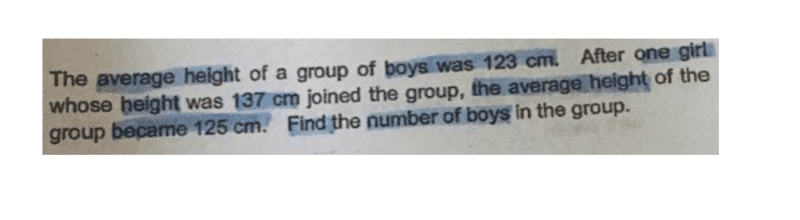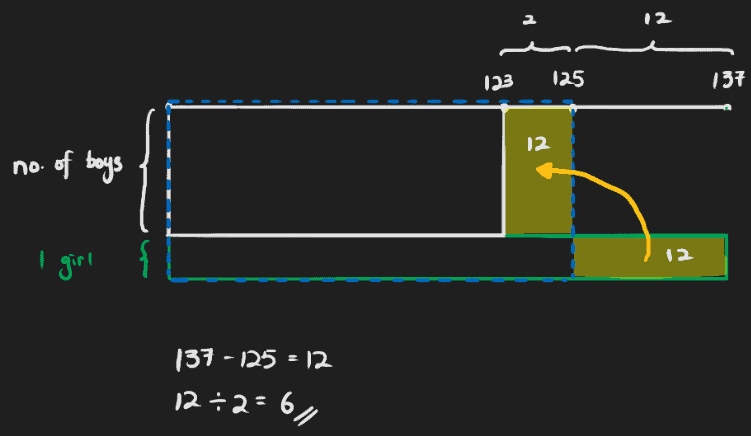# QuestionMay I know how to do this question?

6

0 Replies 0 Likes

When student still cannot grasp the models & algebra solutions, maybe this might help:

Imagine, a girl who is 137cm joins a group of boys, and she suddenly ‘lost’ 12cm (137-125) to become 125cm like the boys.

Before she joined, the boys were 123cm each, hence her joining ‘gained’ 2cm for each boy!

The 12cm lost by the girl must be shared equally by the boys to gain 2cm each, hence 12/2 = 6.

0 Replies 1 Like

Hi

Let the number of Boys be say X

123X +137 ( when the new girl joined ) = 125 (X +1)

123x + 137 = 125x + 125

2x = 14

x =6

Numer of boys is 6 .

Lawrence educator for MOE teachers.

97730543 / www.121tuition.com.sgThink of the area of the boxes as representing the total height of a group.

The top left box is the total for the boys (however many there are).

The green box at the bottom is for that one girl who is 137 cm tall.

The box dotted-line box is the new total for the boys and girl.

This means the extra tail bit from the girl should be added to the missing piece to complete the total. Since both must have an area of 12, it should be easy to figure out that there are 6 boys.

This can be a difficult method to grasp just by looking at it once though…

I can only do this by algebra.

Let s = sum of heights of boys
Let n = number of boys

So, (1) s/n = 123

When girl joins group, we get
(s + 137) / (n+1) = 125
(s + 137) = 125n + 125
Therefore, (2) s = 125n – 12

Substituting (2) into (1),
(125n – 12) / n = 123
(125n – 12) = 123n
2n = 12
so n = 6 #

Someone else should be able to do this by models.

0 Replies 1 Like